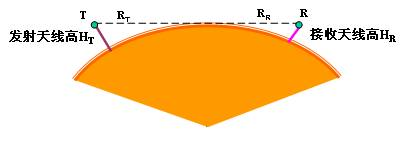## 电磁波的传播

### 移动通信无线电波的传播

• 视距传播• 多径传播

• 绕射传播

### 电磁波的衰减

• 自由空间衰减Free space loss

• 大气吸收Gaseous absorption

• 雨致衰减Rain attenuation

$$\alpha (R) =a R^b dB/km$$
>     $$a(f)=4.09\times 10^{-2}f^{0.699}$$
>     $$b(f)=2.63f^{-0.272}$$
• 云致衰减Cloud attenuation

$$\alpha = 0.4343 \left(\frac{3 \pi \upsilon }{32 \lambda \rho} \right) Im \left(\frac{1-\varepsilon }{2+\varepsilon } \right) dB/km$$
>     $$\upsilon$$ 云层中的液态水含量，$$g/m^3$$；
>     $$\lambda$$ 波长，$$m$$；
>     $$\rho$$ 水的密度，$$g/cm^3$$；
>     $$\varepsilon$$ 水的相对介电常数；
>     $$Im$$ 与云层相关的因子。

• 聚焦和散焦Focusing and defocusing

• 增益下降Decrease in antenna gain due to wave-front incoherence

• 大气闪烁Scintillation

• 多径效应Multipath effects

• 噪声温度Noise temperature

• 去极化Depolarization